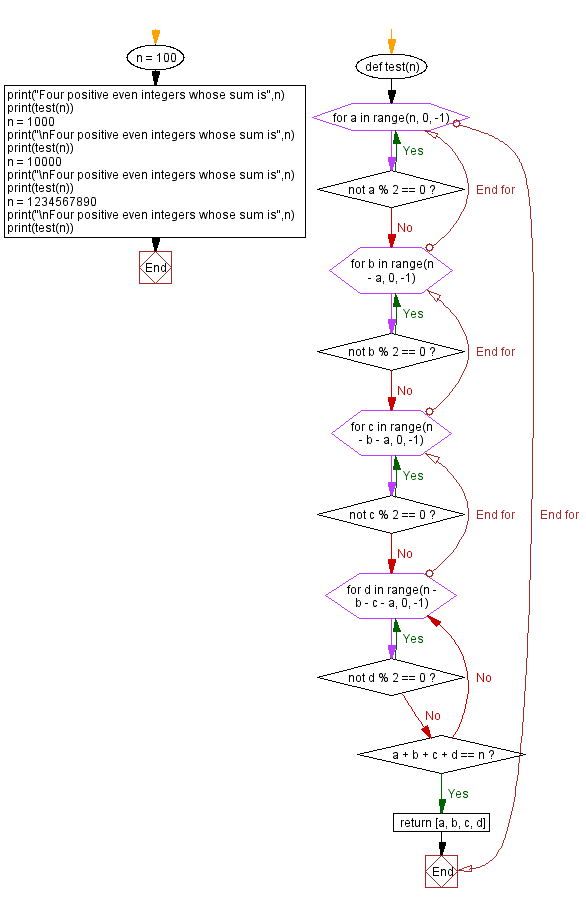﻿ Python: Find four positive even integers whose sum is n - w3resource# Python: Find four positive even integers whose sum is n

## Python Programming Puzzles: Exercise-100 with Solution

Write a Python program to find four positive even integers whose sum is a given integer.

```Input:
n = 100
Output:
[94, 2, 2, 2]

Input:
n = 1000
Output:
[994, 2, 2, 2]

Input:
n = 10000
Output:
[9994, 2, 2, 2]

Input:
n = 1234567890
Output:
[1234567884, 2, 2, 2]
```

Sample Solution:

Python Code:

``````#License: https://bit.ly/3oLErEI

def test(n):
for a in range(n, 0, -1):
if not a % 2 == 0:
continue
for b in range(n - a, 0, -1):
if not b % 2 == 0:
continue
for c in range(n - b - a, 0, -1):
if not c % 2 == 0:
continue
for d in range(n - b - c - a, 0, -1):
if not d % 2 == 0:
continue
if a + b + c + d == n:
return [a, b, c, d]

n = 100
print("Four positive even integers whose sum is",n)
print(test(n))
n = 1000
print("\nFour positive even integers whose sum is",n)
print(test(n))
n = 10000
print("\nFour positive even integers whose sum is",n)
print(test(n))
n = 1234567890
print("\nFour positive even integers whose sum is",n)
print(test(n))
``````

Sample Output:

```Four positive even integers whose sum is 100
[94, 2, 2, 2]

Four positive even integers whose sum is 1000
[994, 2, 2, 2]

Four positive even integers whose sum is 10000
[9994, 2, 2, 2]

Four positive even integers whose sum is 1234567890
[1234567884, 2, 2, 2]
```

Flowchart:## Visualize Python code execution:

The following tool visualize what the computer is doing step-by-step as it executes the said program:

Python Code Editor :

Have another way to solve this solution? Contribute your code (and comments) through Disqus.

Previous: Expand Spaces.

What is the difficulty level of this exercise?

Test your Programming skills with w3resource's quiz.

﻿

## Python: Tips of the Day

Clamps num within the inclusive range specified by the boundary values x and y:

Example:

```def tips_clamp_num(num,x,y):
return max(min(num, max(x, y)), min(x, y))
print(tips_clamp_num(2, 4, 6))
print(tips_clamp_num(1, -1, -6))
```

Output:

```4
-1
```